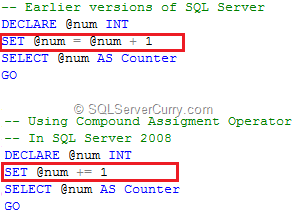### Compound Assignment Operators in SQL Server 2008

Compound assignment operators are newly introduced in SQL Server 2008 and the code certainly looks cleaner and easier to type while using them. I am surprised why weren’t they introduced in earlier SQL Server versions at the first place, however now that they are here, let’s quickly learn how to use them:

Observe the following piece of codeAs you can see, we have used a compound assignment operator to simplify the syntax of adding 1 to the existing value of @num and assigning the result to @num. Although this may look as it is not needed, it is extremely useful where you have T-SQL code which does many arithmetic operations on variables.

Here are a couple of other Compound Assignment Operators for your reference:

-=    Subtract
*=    Multiply
/=    Divide
^=    Bitwise XOR
|=    Bitwise OR
%=   Modulo
&=   Bitwise AND